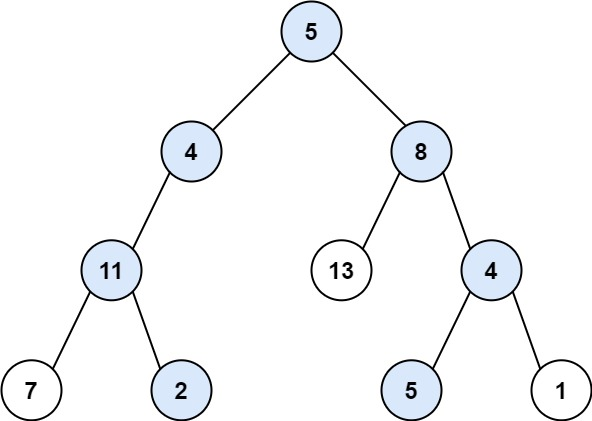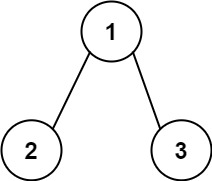304 North Cardinal St.
Dorchester Center, MA 02124

# Path Sum II LeetCode Solution

## Problem – Path Sum II LeetCode Solution

Given the `root` of a binary tree and an integer `targetSum`, return all root-to-leaf paths where the sum of the node values in the path equals `targetSum`. Each path should be returned as a list of the node values, not node references.

root-to-leaf path is a path starting from the root and ending at any leaf node. A leaf is a node with no children.

Example 1:``````Input: root = [5,4,8,11,null,13,4,7,2,null,null,5,1], targetSum = 22
Output: [[5,4,11,2],[5,8,4,5]]
Explanation: There are two paths whose sum equals targetSum:
5 + 4 + 11 + 2 = 22
5 + 8 + 4 + 5 = 22
``````

Example 2:``````Input: root = [1,2,3], targetSum = 5
Output: []
``````

Example 3:

``````Input: root = [1,2], targetSum = 0
Output: []
``````

Constraints:

• The number of nodes in the tree is in the range `[0, 5000]`.
• `-1000 <= Node.val <= 1000`
• `-1000 <= targetSum <= 1000`

## Path Sum II LeetCode Solution in Java

``````    public List<List<Integer>> pathSum(TreeNode root, int sum) {
List<List<Integer>> list = new ArrayList<>();
if (root == null) return list;
List<Integer> path = new ArrayList<>();
Stack<TreeNode> s = new Stack<>();
// sum along the current path
int pathSum = 0;
TreeNode prev = null;
TreeNode curr = root;
while (curr != null || !s.isEmpty()){
// go down all the way to the left leaf node
// add all the left nodes to the stack
while (curr != null){
s.push(curr);
// record the current path
// record the current sum along the current path
pathSum += curr.val;
curr = curr.left;
}
// check left leaf node's right subtree
// or check if it is not from the right subtree
// why peek here?
// because if it has right subtree, we don't need to push it back
curr = s.peek();
if (curr.right != null && curr.right != prev){
curr = curr.right;
continue; // back to the outer while loop
}
// check leaf
if (curr.left == null && curr.right == null && pathSum == sum){
// why do we need new arraylist here?
// if we are using the same path variable path
// path will be cleared after the traversal
}
// pop out the current value
s.pop();
prev = curr;
// subtract current node's val from path sum
pathSum -= curr.val;
// as this current node is done, remove it from the current path
path.remove(path.size()-1);
// reset current node to null, so check the next item from the stack
curr = null;
}
return list;
}
``````

## Path Sum II LeetCode Solution in C++

``````class Solution {
public:
void getAllPaths(TreeNode *root,int targetSum,vector<int> temp,vector<vector<int>> &ans)
{
if(!root)
return;
if(!root->left and !root->right and targetSum == root->val)
{
temp.push_back(root->val);
ans.push_back(temp);
return;
}
temp.push_back(root->val);
getAllPaths(root->left,targetSum-root->val,temp,ans);
getAllPaths(root->right,targetSum-root->val,temp,ans);
}
vector<vector<int>> pathSum(TreeNode* root, int targetSum)
{
vector<vector<int>> ans;
getAllPaths(root,targetSum,{},ans);
return ans;
}
};
``````

## Path Sum II LeetCode Solution in Python

``````class Solution:
def pathSum(self, root, sm):
def dfs(node, sm):
if not node: return []
if not node.left and not node.right and sm == node.val:
return [[node.val]]

lft = dfs(node.left, sm - node.val)
rgh = dfs(node.right, sm - node.val)
return [cand + [node.val] for cand in lft + rgh]

return [s[::-1] for s in dfs(root, sm)]
``````
##### Path Sum II LeetCode Solution Review:

In our experience, we suggest you solve this Path Sum II LeetCode Solution and gain some new skills from Professionals completely free and we assure you will be worth it.

If you are stuck anywhere between any coding problem, just visit Queslers to get the Path Sum II LeetCode Solution

Find on Leetcode

##### Conclusion:

I hope this Path Sum II LeetCode Solution would be useful for you to learn something new from this problem. If it helped you then don’t forget to bookmark our site for more Coding Solutions.

This Problem is intended for audiences of all experiences who are interested in learning about Data Science in a business context; there are no prerequisites.

Keep Learning!

More Coding Solutions >>

LeetCode Solutions

Hacker Rank Solutions

CodeChef Solutions